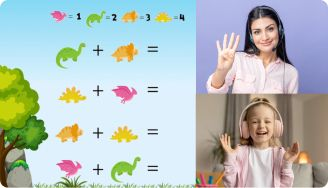# Adding and Subtracting Decimals Worksheets

Addition and subtraction of decimals are more challenging for kids than operations with natural numbers. Luckily, decimal addition and subtraction worksheets have helped many children understand the topic. These worksheets consist of colorful and attractive pages to capture kids’ attention and help them learn.

## Free Addition and Subtraction Decimal Worksheets

Brighterly’s worksheets are excellent manuals for students to practice adding and subtracting decimals. These math worksheets are valuable because addition and subtraction of decimals are an essential part of some math topics kids will face in their academic journey. These interactive worksheets are kids-friendly, which helps students master the concept faster.

Leave your email and we will send you worksheets

worksheets sent successfullyView worksheetView worksheet

## Benefits of Addition and Subtraction of Decimals Worksheets

Generally, it takes time for children to master arithmetic concepts. However, you can help your kid get more comfortable with math operations by providing them with worksheets that may be used more than once. Adding and subtracting decimal worksheets include illustrations and catchy graphics to boost your kid’s desire to learn.

Children like fun activities, and they will jump at the chance to solve math worksheets packed with puzzles and colorful items. Adding and subtracting decimals worksheets will encourage your children to participate more actively in learning activities. In other words, with these worksheets, you will involve your kids and make them learn in a fun and engaging way.

1:1 Math Lessons

Want to raise a genius?
Start learning Math with BrighterlyAdding and subtracting decimal worksheets contain questions of different levels of complexity to boost kids’ understanding. The numbers in worksheets may contain one or more decimal places, which enables kids to learn the topic progressively.

Furthermore, these worksheets help students understand adding and subtracting decimal values. They provide step-by-step solutions to math problems, allowing children to learn and put their knowledge into practice daily.

The primary goal of designing such worksheets is to ensure that students understand and enjoy solving problems with decimals. The use of Brighterly’s add and subtract decimals worksheet will enhance your kid’s problem-solving, critical thinking, and decision-making skills.

Leave your email and we will send you worksheets

worksheets sent successfully

## Printable Addition and Subtraction Worksheets

To master decimals addition and subtraction, kids must study continuously under a tutor’s or parent’s guidance. Brighterly’s adding and subtracting decimals worksheets in PDF help kids learn because you can print them indefinitely. Also, these worksheets come with solutions to problems to help kids learn independently.

### More Decimals Worksheets

Book 1 to 1 Math Lesson• Specify your child’s math level
• Get practice worksheets for self-paced learning
• Your teacher sets up a personalized math learning plan for your childBook 1 to 1 Math Lesson